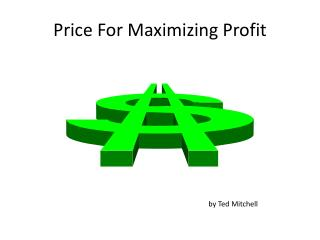# Price For Maximizing Profit - PowerPoint PPT PresentationDownload PresentationPrice For Maximizing Profit

Price For Maximizing ProfitDownload Presentation## Price For Maximizing Profit

- - - - - - - - - - - - - - - - - - - - - - - - - - - E N D - - - - - - - - - - - - - - - - - - - - - - - - - - -
##### Presentation Transcript

1. Price For Maximizing Profit by Ted Mitchell

2. Learning Goal • Finding the Price that Maximizes the Profit is not necessarily the same as finding the Price that Maximizes Revenue

3. Revenue - total Variable Cost - Fixed Cost = Profit Revenue – Total COGS – Total Fixed = Z PQ - VQ - F = Z Z = PQ - VQ - F And Quantity Sold is a function of Selling Price

4. Expand The Basic Profit Equation Z = PQ - VQ - F substitute Q = ƒ(P) = a - bP Z = P(a-bP) - V(a-bP) - F Z = aP - bP2 - aV + bPV - F

5. Z = aP - bP2 - aV + bPV - F Consider the Fixed Costs

6. Z = aP - bP2- aV + bPV - F Consider the Variable Costs Consider the Fixed Costs

7. Z = aP - bP2- aV + bPV - F Consider the Revenue Consider the Variable Costs Consider the Fixed Costs

8. Revenue looks likeR = aP - bP2With zero costs Revenue = Profit Revenue = Profit 0 Price

9. Subtract Fixed Costs from RevenueR - F = aP - bP2 - F Revenue = Profit 0 P* Price

10. Subtract Variable Costs from RevenueR - VQ - F = aP - bP2 - aV + bPV- F Revenue = Profit 0 P* Price P*

11. With NO variable cost per unit, V = 0AndOnly fixed or period costs, F > 0 • If you find the price that maximizes revenue, then you have found the price that maximizes profit. • Price that maximizes revenue is Pr* = a/2b

12. Subtract Variable Costs from RevenueR - VQ - F = aP - bP2 - aV + bPV- F Revenue = Profit 0 P* Price Breakeven Points P*

13. Slope of Revenue Curve is Zero Revenue R 0 P Price

14. Slope of Profit Curve is Zero Profit Z 0 P Price

15. Example Exam Question • The Demand is estimated by market research to be • Q = 5,000 – 500P • The variable cost per unit is, V = \$2 • The fixed cost for the period is, F = \$7,000 • What is the selling price that will maximize the Profit? • First build the Profit EquationThe Revenue is R = P(a-bP2) = P(1,500-500P) • The Profit is Z = R – VQ – F • Z = P(1,500-500P) – 2(1,500-500P) – 1,000 • Z = 1,500P – 500P2 -3,000-1000P – 1,000

16. Example Exam Question • The Demand is estimated by market research to beQ = 5,000 – 500PThe variable cost per unit is, V = \$2The fixed cost for the period is, F = \$7,000What is the selling price that will maximize the Profit? • First build the Profit Equation, ZThe Revenue is R = P(a-bP2) = P(5,000-500P) • The Profit is Z = R – VQ – F • Z = P(5,000-500P) – 2(5,000-500P) – 7,000 • Z = 5,000P – 500P2 -10,000+1000P – 7,000

17. Example Exam Question • The Demand is estimated by market research to beQ = 5,000 – 500PThe variable cost per unit is, V = \$2The fixed cost for the period is, F = \$7,000What is the selling price that will maximize the Profit? • Second: Find the first derivative wrt P, • Z = 5,000P – 500P2 -10,000 + 1,000P – 7,000 • dZ/dP = 5,000 –2(500)P +1,000, set dZ/dP= 0 • 5,000 –2(500)P +1,000 = 0 , solve for P • –2(500)P = -5,000 -1,000 = P = 6,000/1,000 = \$6

18. Revenue Profit \$ \$5 0 \$6 Price

19. There is a General Solution for Finding Optimal Price for Max Profit • 1) Establish the Profit equation • Z = aP - bP2 - aV + bPV – F • 2) Find the first derivative of the profit equation • dZ/dP = a – 2bP – bV • 3) Set the first derivative equal to zero • dZ/dP = a – 2bP – bV = 0 • 4) Solve for the optimal price • P = a/2b + bV/2b = a/2b + V/2b

20. The Price That Maximizes Profit Consider Market Potential Consider Your Variable Costs Consider The Customer’s Sensitivity to Price Changes

21. The Price That Maximizes Profit P = (Price that maximizes revenue) + (Half of the Variable Cost)

22. The Price That Maximizes Profit Is always equal to or higher than the price that maximizes sales revenue!

23. Quantity Maximum Revenue Revenue for Maximum Profit Pr* = \$5 Price Pz* = \$6

24. Quantity Maximum Revenue Revenue for Maximum Profit a/2 Pr* = a/2b Price Pz* = a/2b + V/2

25. The Price That Maximizes Profit Says if you get an increase in your variable costs pass half of it on to the customer.

26. The Price That Maximizes Profit Note: It Says Do NOT change your price just because you get an increase in your fixed costs!

27. Any Questions?